# Texas Go Math Grade 8 Lesson 5.3 Answer Key Linear Relationships and Bivariate Data

Refer to our Texas Go Math Grade 8 Answer Key Pdf to score good marks in the exams. Test yourself by practicing the problems from Texas Go Math Grade 8 Lesson 5.3 Answer Key Linear Relationships and Bivariate Data.

## Texas Go Math Grade 8 Lesson 5.3 Answer Key Linear Relationships and Bivariate Data

Essential Question
How can you contrast linear and nonlinear sets of bivariate data?

Find the equation of each linear relationship.

Question 1.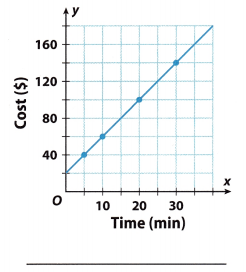Slope = $$\frac{60-40}{10-5}$$ = $$\frac{20}{5}$$ = 4
Find the slope using two points from the graph by Slope(m) = $$\frac{y_{2}-y_{1}}{x_{2}-x_{1}}$$ where (x2, y2) = (10, 60) and (x1, y1) = (5, 40)
b = 20 Read the y-intercept from the graph (when x = 0)
y = 4x + 20 Substituting the value of slope (m) and y intercept in slope-intercept form.
y = mx + b
y = 4x + 20

Question 2.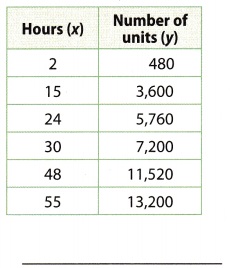Slope = $$\frac{3600-480}{15-2}$$ = $$\frac{3120}{13}$$ = 240
Find the slope using two points from the graph by Slope(m) = $$\frac{y_{2}-y_{1}}{x_{2}-x_{1}}$$ where (x2, y2) = (15, 3600) and (x1, y1) = (2, 480)
b = 20 Read the y-intercept from the graph (when x = 0)y = 240x Substituting the value of slope (m) and y intercept in slope-intercept form. y = mx + b
y = 240x

Reflect

Question 3.
What If? Suppose a regulation changes the cost of the taxi ride to $1.80 per mile, plus a fee of$4.30. How does the price of the 6.5-mile ride compare to the original price?
y = 1.8x + 4.3 Substituting the value of slope (m) and y intercept in slope-intercept form.
y = mx + b
where m = 1.8 and b = 4.3

y = 1.8(6.5) + 4.3 Substitute x = 6.5 mile
y = 11.7 + 4.3 = 16
The cost remains the same.

Question 4.
How can you use a graph of a linear relationship to predict a value for a new input?
Use the two points from the graph to determine the slope. Determine the y intercept from the graph Substitute the m and b in slope-intercept form: y = mx + b
Substitute x in the equation to find y.

Question 5.
How can you use a table of linear data to predict a value?
Use the two points from the table to determine the slope. Determine the y intercept from slope and given point by substituting in slope-intercept form: y = mx + b
Write the equation. Substitute x in the equation to find y.

Paulina’s income from a job that pays her a fixed amount per hour is shown in the graph. Use the graph to find the predicted value.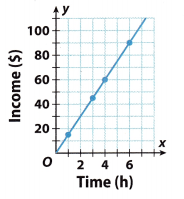Question 6.
Income earned for working 2 hours ______
From the graph we can read that in 2 hours, her income is 30.Butlet’strytogetitbycalculatingtheslopeand y$-intercept From the graph, we can see that the line passes through the origin, which means that this linear relationship is proportional. Therefore our starting point is (0, 0), and what else we can conclude is that our y-intercept (b) in this case is 0. → b = 0 The slope is calculated by: m = $$\frac{y_{2}-y_{1}}{x_{2}-x_{1}}$$ Choose two points from the graph and put them into the calculation. I’ll take (4, 60) for (x1, y1) and (6, 90) for (x2, y2). Thus m = $$\frac{90-60}{6-4}$$ m = $$\frac{30}{2}$$ m = 15 We need to find the value of y, income earned in for working 2hours. x represents the time in hours. The standard form of the linear equation is y = mx + b, so include the obtained values of x, the slope, and y-intercept. y = 15 . 2 +0 y = 30 + 0 y = 30 To conclude, for 2 hours of work Paulina gets$30

Question 7.
Income earned for working 3.25 hours ______
From the graph, we can see that the line passes through the origin, which means that this linear relationship is proportional.
Therefore our starting point is (0, 0), and what else we can conclude is that our y-intercept (b) in this case is 0. → b = 0
The slope is calculated by:
m = $$\frac{y_{2}-y_{1}}{x_{2}-x_{1}}$$
Choose two points from the graph and put them into the calculation. I’ll take (4, 60) for (x1, y1) and (6, 90) for (x2, y2). Thus
m = $$\frac{90-60}{6-4}$$
m = $$\frac{30}{2}$$
m = 15

We need to find the value of y, income earned in for working 3.5 hours. x represents the time in hours. The standard form of the linear equation is y = mx + b, so include the obtained values of x, the slope, and y-intercept.
y = 15 . 3.5 + 0
y = 52.5 + 0
y = 52.5
$To conclude, for 3.5 hours of work Paulina gets$52.5

Question 8.
Total income earned for working for five 8-hour days all at the standard rate ______
Income after 8 hr = $120 Estimated from the graph, when x = 8hrs Income after 5 – 8hrs = 120 * 5 =$600

Contrasting Linear and Nonlinear Data
Bivariate data is a set of data that is made up of two paired variables. If the relationship between the variables is linear, then the rate of change (slope) is constant. If the graph shows a nonlinear relationship, then the rate of change varies between pairs of points.

Andrew has two options in which to invest $200. Option A earns simple interest of 5%, while Option B earns interest of 5% compounded annually. The table shows the amount of the investment for both options over 20 years. Graph the data and describe the differences between the two graphs.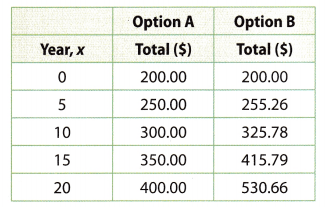Step 1 Graph the data from the table for Options A and B on the same coordinate grid.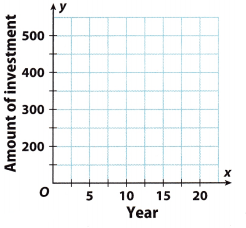Step 2 Find the rate of change between pairs of points for Option A and classify the relationship.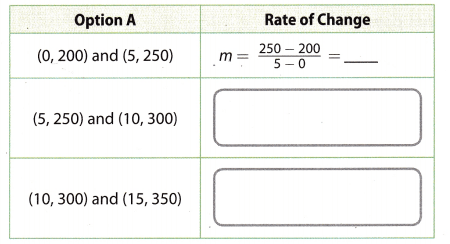The rate of change between the data values is ____, so the graph of Option A shows a __________ relationship. Step 3 Find the rate of change between pairs of points for Option B and classify the relationship.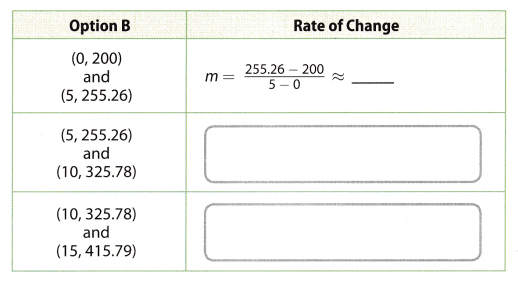The rate of change between the data values is ____, so the graph of Option B shows a ____ relationship. Reflect Question 9. Why are the graphs drawn as lines or curves and not discrete points? Answer: The number of years can be in fraction, hence graph are drawn as lines or curves and not discrete points Question 10. Can you determine by viewing the graph if the data have a linear or nonlinear relationship? Explain. Answer: Yes. Linear relationship is represented by a straight line and non-linear relationship is represented by a curve. Question 11. Draw Conclusions Find the differences in the account balances to the nearest dollar at 5-year intervals for Option B. Flow does the length of time that money is in an account affect the advantage that compound interest has over simple interest? Answer: Difference (0 – 5) = 255.26 – 200 = 55.26 Difference (5 – 10) = 325.78 – 255.26 = 70.52 Difference (10 – 15) = 415.79 – 325.78 = 90.01 Difference (15 – 20) = 530.66 – 415.79 = 114.87 The amount of interest earned increases with the number of years for compound interest. Texas Go Math Grade 8 Lesson 5.3 Guided Practice Answer Key Use the following graphs to find the equation of the linear relationship. (Example 1) Question 1.Answer: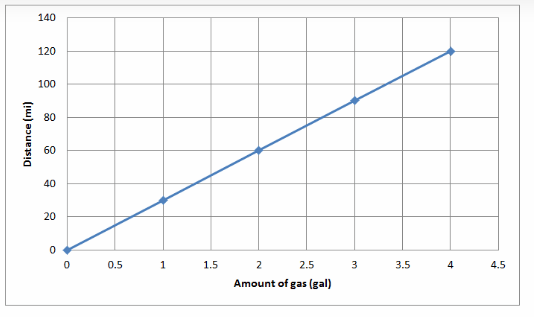Use the given points to show that it is a linear relationship Slope = $$\frac{60-30}{2-1}$$ = $$\frac{30}{1}$$ = 30 Find the slope using two points from the graph by Slope(m) = $$\frac{y_{2}-y_{1}}{x_{2}-x_{1}}$$ where (x2, y2) = (2, 60) and (x1, y1) = (1, 30) b = 0 Read the y-intercept from the graph (when x = 0) y = 30x Substituting the value of slope (m) and y intercept in slope-intercept form. y = mx + b y = 30x Question 2.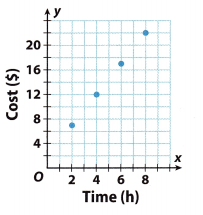Answer: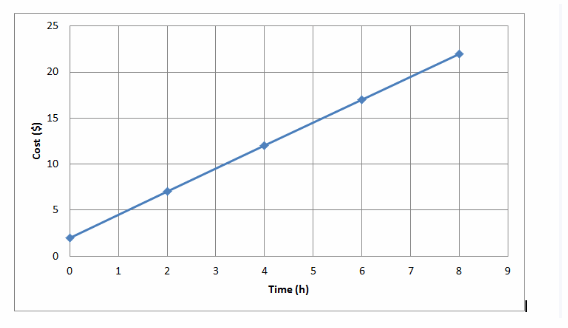Use the given points to show that it is a linear relationship. Slope = $$\frac{12-7}{4-2}$$ = $$\frac{5}{2}$$ = 2.5 Find the slope using two points from the graph by Slope(m) = $$\frac{y_{2}-y_{1}}{x_{2}-x_{1}}$$ where (x2, y2) = (4, 12) and (x1, y1) = (2, 7) b = 2 Read the y-intercept from the graph (when x = 0) y = 2.5x + 2 Substituting the value of slope (m) and y intercept in slope-intercept form. y = mx + b y = 2.5x + 2 Question 3. The graph shows the relationship between the number of hours a kayak is rented and the total cost of the rental. Write an equation of the relationship. Then use the equation to predict the cost of a rental that lasts 5.5 hours. (Example 2)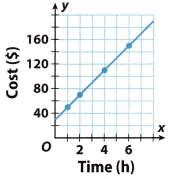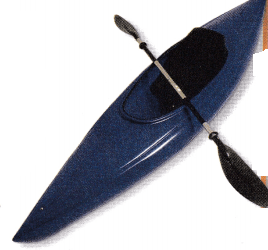Answer: Write the equation of the linear relationship. (2, 70) and (4, 110)(Select two points) m = $$\frac{110-70}{4-2}$$ (Calculate the rate of change) = $$\frac{40}{20}$$ (Simplify) = 20 y = mx + b 70 = 20(2) + b (Fill in values for x, y and m) 70 = 40 + b (Simplify) 30 = b (Solve for b) The equation of nie linear relationship is y = 20x + 30. Use your equation from Step 1 to predict the cost of rental that last 5.5 hours. y = 20x + 30 (Substitute x=5.5) y = 20(5.5) + 30 y = 110 + 30 (Solve for y) y = 140 The cost of a rental that last 5.5 hours is \140$.

Does each of the following tables represent a linear relationship? Why or why not? (Explore Activity)

Question 4.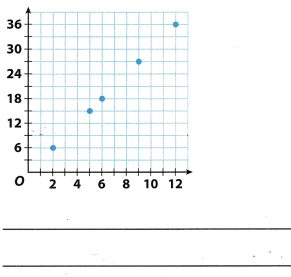Yes The graph has a constant rate of change

Question 5.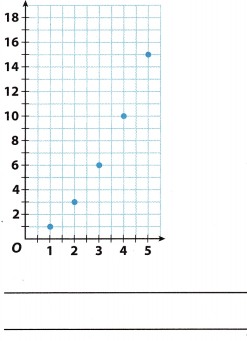No
The graph does not have a constant rate of change

Essential Question Chk-In

Question 6.
How can you tell ¡fa set of bivariate data shows a linear relationship?
It is a linear relationship if the rate of change is constant or the graph is a straight line

Does each of the following tables represent a linear relationship? Why or why not?

Question 7.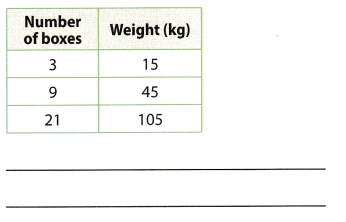Rate of change = $$\frac{45-15}{9-3}$$ = $$\frac{30}{6}$$ = 5 Find the rate of change using
Rate of change = $$\frac{105-45}{21-9}$$ = $$\frac{60}{12}$$ = 5
Slope (m) = $$\frac{y_{2}-y_{1}}{x_{2}-x_{1}}$$
It is a linear relationship as the rate of change is constant.

Question 8.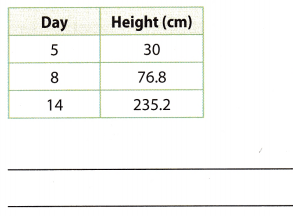Rate of change = $$\frac{76.8-30}{8-5}$$ = $$\frac{46.8}{3}$$ = 15.6 Find the rate of change using Slope (m) = $$\frac{y_{2}-y_{1}}{x_{2}-x_{1}}$$
Rate of change = $$\frac{235.2-76.8}{14-8}$$ = $$\frac{158.4}{6}$$ = 26.4
It is a linear relationship as the rate of change is constant.

Explain whether or not you think each relationship is linear.

Question 9.
the cost of equal-priced DVDs and the number purchased
Explanation A:
the relation between the cost of equal-priced DVDs and the number purchased is linear, because the change rate
is constant. If we denote with e the cost of a DVD, with x the number of purchased DVDs and with y the total cost of the purchased DVDs, we get an equation of the form:
y = cx

Explanation B:
Linear relationship The cost of DVDs increases at a constant rate with number of purchases.

Question 10.
the height of a person and the person’s age
Non – Linear relationship The height of a person does not increase at a constant rate with person’s age

Question 11.
the area of a square quilt and its side length
We can say that a relationship is linear if the rate of change or the slope is constant

In the relationship of the area of a square quilt and its side length, the independent variable is the side length and
the dependent variable is the area. Now, say for example, the side of the quilt is 4 feet 3 feet 2 feet its length will
be 42, 32 and 22 square feet, respectively.

Now, we have these set of ordered pairs: {(2, 4), (3, 9), (4, 16)}. Based on this example, we have the following as possible solution for the slope:
when we use {(2, 4), (3, 9)}, slope is
$$\frac{9-4}{3-2}[latex] = 5 when we use {(3, 9), (4, 16)}, slope is [latex]\frac{16-9}{4-3}[latex] = 7 Looking at the slope, they are not constant, as the area and the sides increases, the rate of change also increases, thus, this is a nonlinear relationship See the explanation. Question 12. the number of miles to the next service station and the number of kilometers Answer: We can say that a relationship is linear if the rate of change or the slope is constant. In the relationship of the number of miles to the next service station and the number of kilometers, the independent variable is number of kilometers and the dependent variable is the number of miles. Now, for these values of the number of kilometers, {1, 2, 3, 4}, the corresponding number of miles are: {0.621, 1.21, 1.86, 2.485} when we use {(1, 0.61), (2, 1.243)}, slope is [latex]\frac{1.24-0.62}{2-1}$$ = 0.62
when we use {(3, 1.86), (4, 2.48)}, slope is
$$\frac{2.48-1.86}{4-3}$$ = 0.62
Looking at the slope, they are constant, as the area and the sides increases, the rate of change remains the same, thus, this is a linear relationship

See the explanation.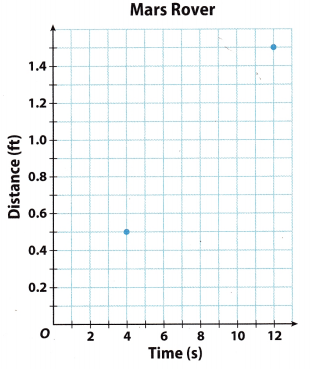Question 13.
Multistep The Mars Rover travels 0.75 feet in 6 seconds. Add the point to the graph. Then determine whether the relationship between distance and time is linear, and if so, predict the distance that the Mars Rover would travel in 1 minute.
The black points on the chart are the default. The green point is what we were supposed to plotTo see if our linear relationship ¡s proportional, calculate the rate of change from the given information. To know if some linear relationship is proportional or nonproportional, we have to use ratio m = $$\frac{y}{x}$$, which needs to be constant for proportionality.

We have 3 points on the graph: (4, 0.5); (6, 0.75) and (12, 1.5). Put those in the calculation:
m = $$\frac{0.5}{4}$$ = 0.125
m = $$\frac{0.75}{6}$$ = 0.125
m = $$\frac{1.5}{12}$$ = 0.125

The rate of change is constant so this relationship is proportional. If the relationship is proportional we can
immediately conclude that our y-intercept is equal to 0 (b = 0).

The standard form of the linear equation is y = mx + b.
Our equation in slope-intercept form is:
y = 0.125x + 0 → y = 0.125x
y represents the distance (ft), and x represents the time (s).

We need to determine the distance that the Mars Rover would travel in 1 minute. Since the time is measured in seconds, we need to convert the minute to the default unit So 1 minute has 60 seconds. Put it in the equation to
get the distance.
y = 0.125 • 60
y = 7.5
In one minute, the Mars Rover would travel 7.5 ft.

Question 14.
Make a Conjecture Zefram analyzed a linear relationship, found that the slope-intercept equation was y = 3.5x + 16, and made a prediction for the value of y for a given value of x. He realized that he made an error calculating they-intercept and that it was actually 12. Can he just subtract 4 from his prediction if he knows that the slope is correct? Explain.
Zefram used y = 3.5x + 16 for the prediction of y for a given value of æ. But eventually he found out that he made an error calculating the y-intercept and that it was actually 12

Now, if he wants to make correction his prediction knowing that the slope is correct, he can simply subtract the
predicted value by 4. Look at these illustrations.
y = 3.5x + 16 → y = (3.5x – 16) 4 → y = 3.5x + 12

Since by subtracting 4 from the predicted value of y would make y-intercept be equal to 12(as shown
in the illustrations), then Zefram can subtract 4 from the predicted value of y. So the answer is Yes.

See the explanation.

Texas Go Math Grade 8 Lesson 5.3 H.O.T. Focus On Higher Order Thinking Answer Key

Question 15.
Communicate Mathematical Ideas The table shows a linear relationship. How can you predict the value of y when x = 6 without finding the equation of the relationship?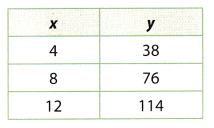The only calculation in which we have values for both x and y is the slope calculation.
Since y is unknown and we have to find its value, first we must calculate the slope to include its value as well in the
calculation. Otherwise, both the slope and y would be unknown.

So, to get the slope, choose two points from the table:
m = $$\frac{y_{2}-y_{1}}{x_{2}-x_{1}}$$
m = $$\frac{114-76}{12-8}$$
m = $$\frac{38}{4}$$
m = 9.5

To get y, when X = 6, choose one point from the table, include it into the calculation, include value of x and the slope.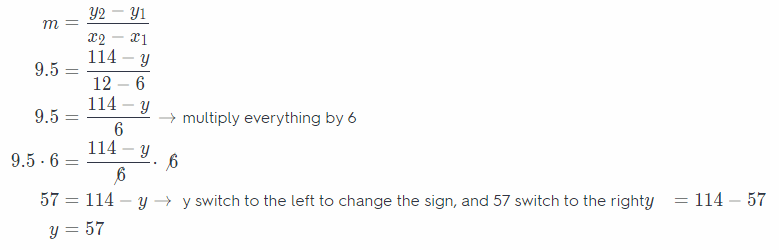The obtained value of y is 57 when x = 6. → (6, 57).

Question 16.
Critique Reasoning Louis says that if the differences between the values of x are constant between all the points on a graph, then the relationship is linear. Do you agree? Explain.
If the relationship between the variables is linear, then the rate of change(slope) is constant The slope is simply the difference between the y values divided by the difference of the corresponding x values.

Louis says that if the differences between the values of x are constant between all the points on a graph, then the relationship is linear. I do not agree with this idea of Louis since some of the values of x may not be with the same difference as the other points. For example, x values of a Linear equation are {1, 3, 7, 8}, the difference between 1 and 3 is different from the difference between 7 and 8, and so on.

See the explanation.

Question 17.
Make a Conjecture Suppose you know the slope of a linear relationship and one of the points that its graph passes through. How could you predict another point that falls on the graph of the line?
Find the equation of the Linear relationship using the slope and given point. Then insert any x-value to find find a y value on the graph of the line.

Question 18.
Explain the Error Thomas used (7, 17.5) and (18, 45) from a graph to find the equation of a linear relationship as shown. What was his mistake?
m = $$\frac{45-7}{18-17.5}$$ = $$\frac{38}{0.5}$$ = 79
y = 79x + b
45 = 79 • 18 + b
45 = 1422 + b, so b = -1377
The equation is y = 79x – 1377.
Thomas used (7, 17.5) and (18, 45) from the graph to find the linear relationship. Let us examine his mistake in his solution below.
m = $$\frac{45-7}{18-17.5}$$ = $$\frac{38}{0.5}$$ = 79
y = 79x + b
45 = 79 • 18 + b
45 = 1422 + b, so b = -1377
The equation is y = 79x – 1377

As we can notice, there is a mistake on the substitution of values of the variables in getting the slope m. The formula in finding the slope is
m = $$\frac{y_{2}-y_{1}}{x_{2}-x_{1}}$$
In the problem, the points are (7, 17.5) and (18, 45), wherein
x1 = 7, y1 = 17.5, x2 = 18, y2 = 45
Instead of substituting 17.5 as the value of y, Thomas substitute 7 and instead of substituting 7 as the value of x1, he substitute 17.5. In short, Thomas interchanged the values of x1 and y1.

Since Thomas made a mistake in the first step of the solution, definitely the proceeding solutions are also incorrect.

Here is the correct answer for the given problem in the statement.
Substituting the values of the variables gives the slope ofSolving for the y-intercept, where in = 2.5 using the point (18, 45)
y = 2.5x + b
45 = 2.5(18)+b
45 = 45 + b
b = 45 – 45
b = 0

Now, having the slope m = 2.5 and y-intercept b = 0, the equation is
y = 2.5x + 0
y = 2.5x

Scroll to Top